## Galois groups of finite fields

Each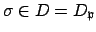acts in a well-defined way on the finite field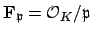, so we obtain a homomorphism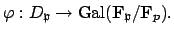We pause for a moment and derive a few basic properties of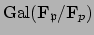, which are in fact general properties of Galois groups for finite fields. Let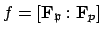.

The group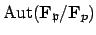contains the element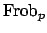defined by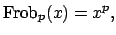because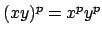and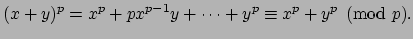By Exercise 29 (see Chapter 22), the group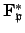is cyclic, so there is an element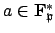of order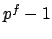, and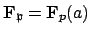. Then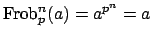if and only if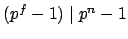which is the case preciselywhen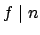, so the order ofis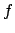. Since the order of the automorphism group of a field extension is at most the degree of the extension, we conclude thatis generated by. Also, sincehas order equal to the degree, we conclude that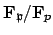is Galois, with groupcyclic of ordergenerated by. (Anther general fact: Up to isomorphism there is exactly one finite field of each degree. Indeed, if there were two of degree, then both could be characterized as the set of roots in the compositum of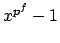, hence they would be equal.)

William Stein 2004-05-06